## 4.3 Rejection sampling

This section deals with a general algorithm for simulating variables from a distribution with density $$f$$. We call $$f$$ the target density and the corresponding distribution is called the target distribution. The idea is to simulate proposals from a different distribution with density $$g$$ (the proposal distribution) and then according to a criterion decide to accept or reject the proposals. It is assumed throughout that the proposal density $$g$$ is a density fulfilling that

$\begin{equation} g(x) = 0 \Rightarrow f(x) = 0. \tag{4.1} \end{equation}$

Let $$Y_1, Y_2, \ldots$$ be i.i.d. with density $$g$$ on $$\mathbb{R}$$ and $$U_1, U_2, \ldots$$ be i.i.d. uniformly distributed on $$(0,1)$$ and independent of the $$Y_i$$-s. Define $T(\mathbf{Y}, \mathbf{U}) = Y_{\sigma}$ with $\sigma = \inf\{n \geq 1 \mid U_n \leq \alpha f(Y_n) / g(Y_n)\},$ for $$\alpha \in (0, 1]$$ and $$f$$ a density. Rejection sampling then consists of simulating independent pairs $$(Y_n, U_n)$$ as long as we reject the proposals $$Y_n$$ sampled from $$g$$, that is, as long as $U_n > \alpha f(Y_n) / g(Y_n).$ The first time we accept a proposal is $$\sigma$$, and then we stop the sampling and return the proposal $$Y_{\sigma}$$. The result is, indeed, a sample from the distribution with density $$f$$ as the following theorem states.

Theorem 4.2 If $$\alpha f(y) \leq g(y)$$ for all $$y \in \mathbb{R}$$ and $$\alpha > 0$$ then the distribution of $$Y_{\sigma}$$ has density $$f$$.

Proof. Note that $$g$$ automatically fulfills (4.1). The formal proof decomposes the event $$(Y_{\sigma} \leq y)$$ according to the value of $$\sigma$$ as follows

\begin{align} P(Y_{\sigma} \leq y) & = \sum_{n = 1}^{\infty} P(Y_{n} \leq y, \ \sigma = n) \\ & = \sum_{n = 1}^{\infty} P(Y_{n} \leq y, \ U_n \leq \alpha f(Y_n) / g(Y_n)) P(\sigma > n - 1) \\ & = P(Y_{1} \leq y, \ U_1 \leq \alpha f(Y_1) / g(Y_1)) \sum_{n = 1}^{\infty} P(\sigma > n - 1). \end{align}

By independence of the pairs $$(Y_n, U_n)$$ we find that $P(\sigma > n - 1) = p^{(n-1)}$ where $$p = P(U_1 > \alpha f(Y_1) / g(Y_1))$$, and $\sum_{n = 1}^{\infty} P(\sigma > n - 1) = \sum_{n = 1}^{\infty} p^{(n-1)} = \frac{1}{1 - p}.$

We further find using Tonelli’s theorem that

\begin{align} P(Y_{1} \leq y, \ U_1 \leq \alpha f(Y_1) / g(Y_1)) & = \int_{-\infty}^y \alpha \frac{f(z)}{g(z)} g(z) \mathrm{d}z \\ & = \alpha \int_{-\infty}^y f(z) \mathrm{d} z. \end{align}

It also follows from this, by taking $$y = \infty$$, that $$1 - p = \alpha$$, and we conclude that $P(Y_{\sigma} \leq y) = \int_{-\infty}^y f(z) \mathrm{d} z,$ and the density for the distribution of $$Y_{\sigma}$$ is, indeed, $$f$$.

Note that if $$\alpha f \leq g$$ for densities $$f$$ and $$g$$, then $\alpha = \int \alpha f(x) \mathrm{d}x \leq \int g(x) \mathrm{d}x = 1,$ whence it follows automatically that $$\alpha \leq 1$$ whenever $$\alpha f$$ is dominated by $$g$$. The function $$g/\alpha$$ is called the envelope of $$f$$. The tighter the envelope, the smaller is the probability of rejecting a sample from $$g$$, and this is quantified explicitly by $$\alpha$$ as $$1 - \alpha$$ is the rejection probability. Thus $$\alpha$$ should preferably be as close to one as possible.

If $$f(y) = c q(y)$$ and $$g(y) = d p(y)$$ for (unknown) normalizing constants $$c, d > 0$$ and $$\alpha' q \leq p$$ for $$\alpha' > 0$$ then $\underbrace{\left(\frac{\alpha' d}{c}\right)}_{= \alpha} \ f \leq g.$ The constant $$\alpha'$$ may be larger than 1, but from the argument above we know that $$\alpha \leq 1$$, and Theorem 4.2 gives that $$Y_{\sigma}$$ has distribution with density $$f$$. It appears that we need to compute the normalizing constants to implement rejection sampling. However, observe that $u \leq \frac{\alpha f(y)}{g(y)} \Leftrightarrow u \leq \frac{\alpha' q(y)}{p(y)},$ whence rejection sampling can actually be implemented with knowledge of the unnormalized densities and $$\alpha'$$ only and without computing $$c$$ or $$d$$. This is one great advantage of rejection sampling. We should note, though, that when we don’t know the normalizing constants, $$\alpha'$$ does not tell us anything about how tight the envelope is, and thus how small the rejection probability is.

Given two functions $$q$$ and $$p$$, how do we then find $$\alpha'$$ so that $$\alpha' q \leq p$$? Consider the function $y \mapsto \frac{p(y)}{q(y)}$ for $$q(y) > 0$$. If this function is lower bounded by a value strictly larger than zero, we can take $\alpha' = \inf_{y: q(y) > 0} \frac{p(y)}{q(y)} > 0.$ We can in practice often find this value by minimizing $$p(y)/q(y)$$. If the minimum is zero, there is no $$\alpha'$$, and $$p$$ cannot be used to construct an envelope. If the minimum is strictly positive it is the best possible choice of $$\alpha'$$.

### 4.3.1 von Mises distribution

Recall the von Mises distribution from Section 1.2.1. It is a distribution on $$(-\pi, \pi]$$ with density $f(x) \propto e^{\kappa \cos(x - \mu)}$ for parameters $$\kappa > 0$$ and $$\mu \in (-\pi, \pi]$$. Clearly, $$\mu$$ is a location parameter, and we fix $$\mu = 0$$ in the following. Simulating random variables with $$\mu \neq 0$$ can be achieved by (wrapped) translation of variables with $$\mu = 0$$.

Thus the target density is $$f(x) \propto e^{\kappa \cos(x)}$$. In this section we will use the uniform distribution on $$(-\pi, \pi)$$ as proposal distribution. It has constant density $$g(x) = (2\pi)^{-1}$$, but all we need is, in fact, that $$g(x) \propto 1$$. Since $$x \mapsto 1 / \exp(\kappa \cos(x)) = \exp(-\kappa \cos(x))$$ attains its minimum $$\exp(-\kappa)$$ for $$x = 0$$, we find that $\alpha' e^{\kappa \cos(x)} = e^{\kappa(\cos(x) - 1)} \leq 1,$ with $$\alpha' = \exp(-\kappa)$$. The rejection test of the proposal $$Y \sim g$$ can therefore be carried out by testing if a uniformly distributed random variable $$U$$ on $$(0,1)$$ satisfies $U > e^{\kappa(\cos(Y) - 1)}.$

vMsim_slow <- function(n, kappa) {
y <- numeric(n)
for(i in 1:n) {
reject <- TRUE
while(reject) {
y0 <- runif(1, - pi, pi)
u <- runif(1)
reject <- u > exp(kappa * (cos(y0) - 1))
}
y[i] <- y0
}
y
}
f <- function(x, k) exp(k * cos(x)) / (2 * pi * besselI(k, 0))
x <- vMsim_slow(100000, 0.5)
hist(x, breaks = seq(-pi, pi, length.out = 20), prob = TRUE)
curve(f(x, 0.5), -pi, pi, col = "blue", lwd = 2, add = TRUE)
x <- vMsim_slow(100000, 2)
hist(x, breaks = seq(-pi, pi, length.out = 20), prob = TRUE)
curve(f(x, 2), -pi, pi, col = "blue", lwd = 2, add = TRUE)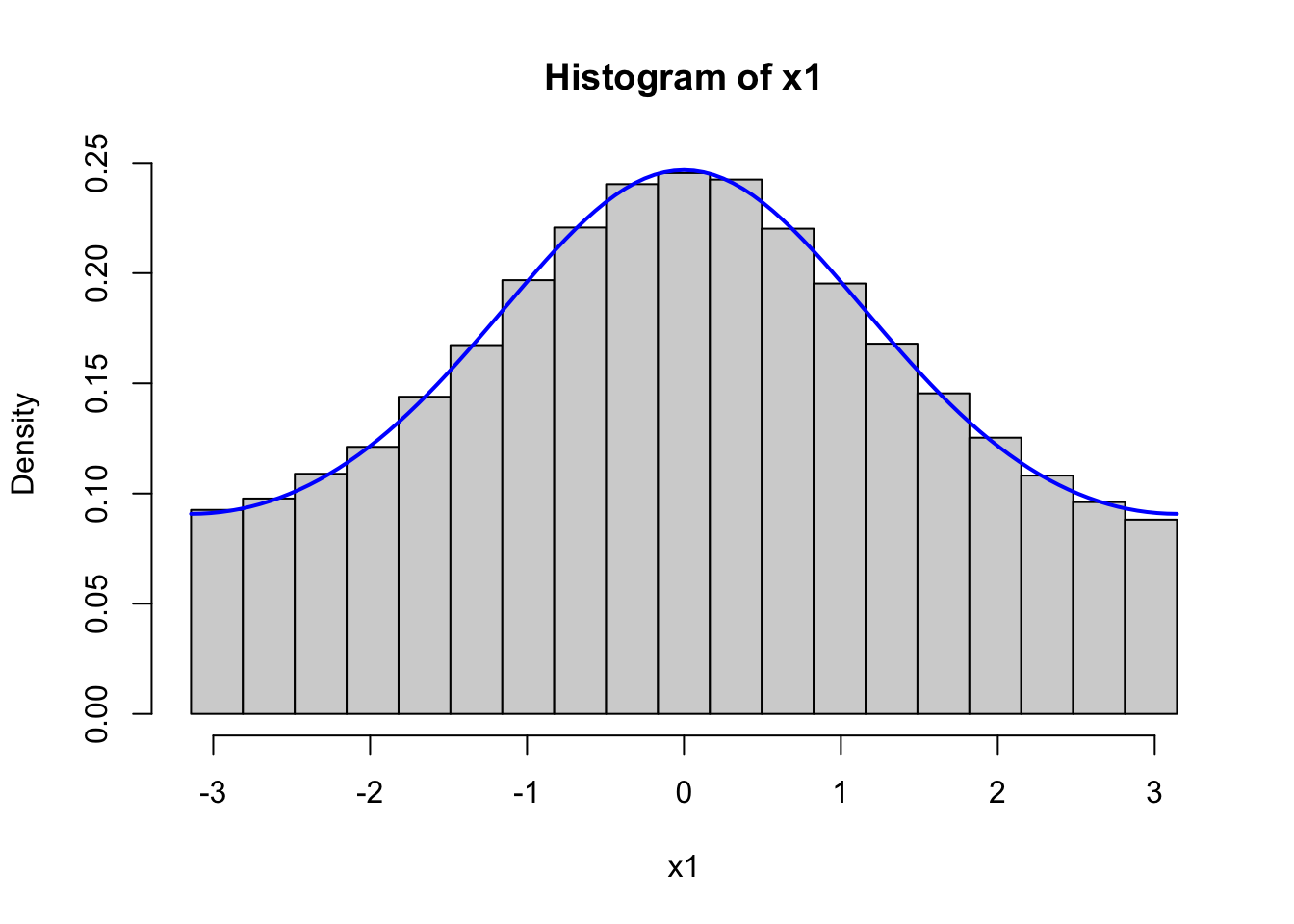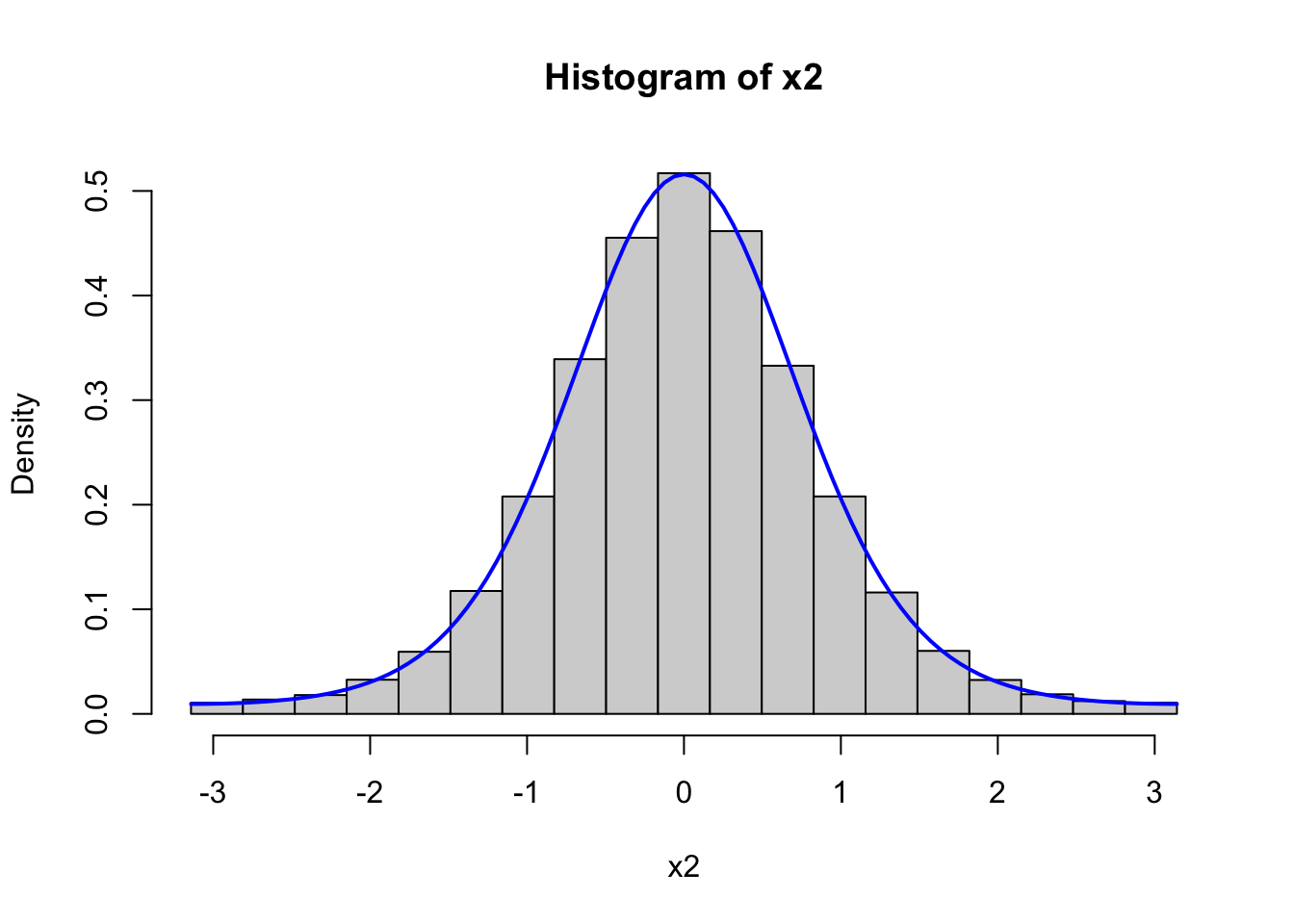Figure 4.1: Histograms of 100,000 simulated data points from von Mises distributions with parameters $$\kappa = 0.5$$ (left) and $$\kappa = 2$$ (right). The true densities (blue) are added to the plots.

Figure 4.1 confirms that the implementation simulates from the von Mises distribution.

system.time(vMsim_slow(100000, kappa = 5))
##    user  system elapsed
##   2.508   0.090   2.608

Though the implementation can easily simulate 100,000 variables in a couple of seconds, it might still be possible to improve it. To investigate what most of the run time is spent on we use the line profiling tool as implemented in the profvis package.

library(profvis)
profvis(vMsim_slow(10000, 5))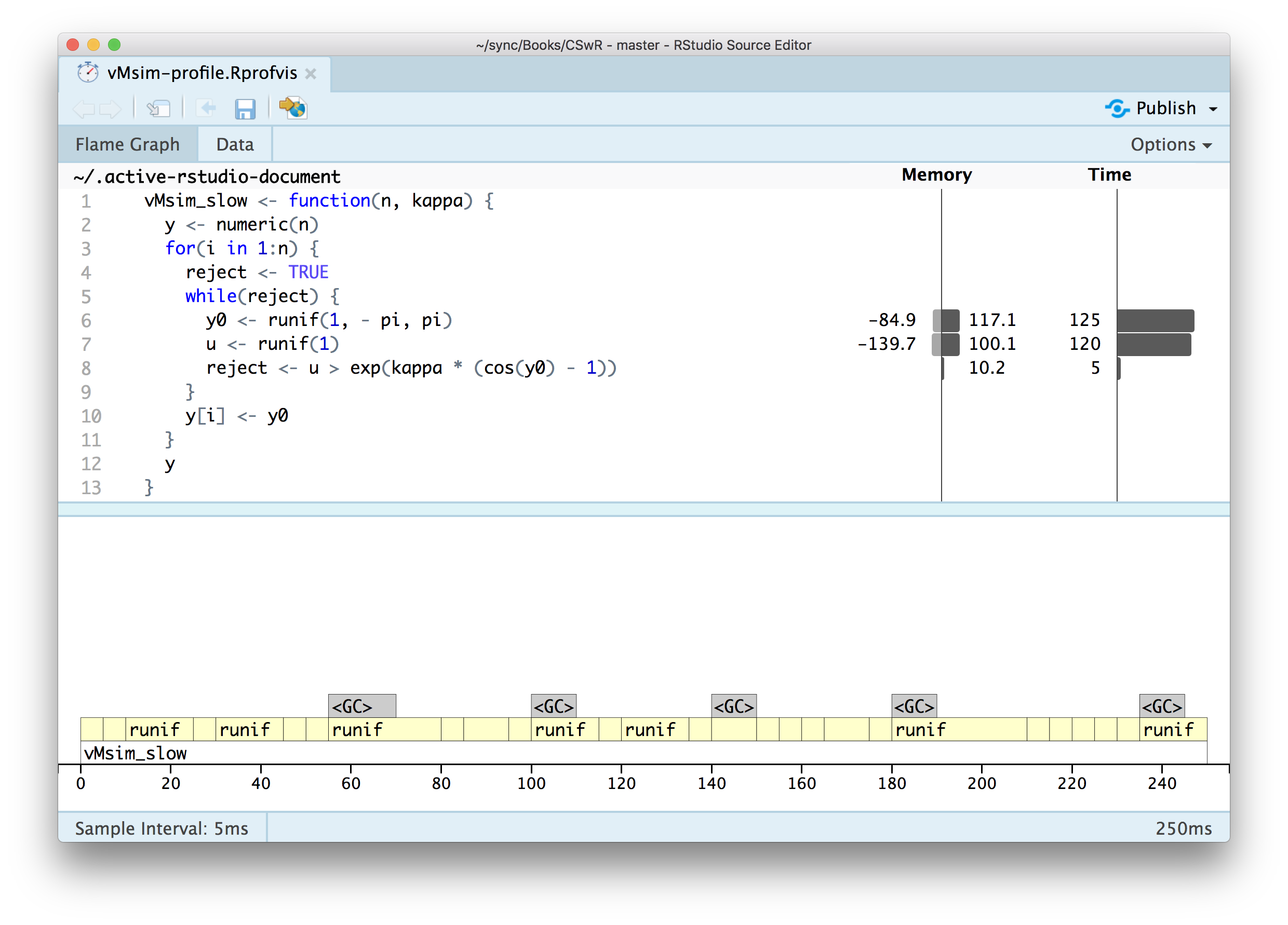The profiling result shows that almost all the time is spent on simulating uniformly distributed random variables. It is, perhaps, expected that this should take some time, but that it takes so much more time than computing the ratio, say, used for the rejection test is a bit surprising. What might be even more surprising is the large amount of memory allocation and deallocation associated with the simulation of the variables.

The culprit is runif that has some overhead associated with each call. The function performs much better if called once to return a vector than if called repeatedly as above to return just single numbers. We could rewrite the rejection sampler to make better use of runif, but it would make the code a bit more complicated because we don’t know upfront how many uniform variables we need. This will introduce some bookkeeping that it is possible to abstract away from the implementation of any rejection sampler. Therefore we implement a generic wrapper of the random number generator that will cache a suitable amount of random variables. This function will take care of some bookkeeping and variables can then be extracted as needed. This also nicely illustrates the use of a function factory.

rng_stream <- function(m, rng, ...) {
args <- list(...)
cache <- do.call(rng, c(m, args))
j <- 0
fact <- 1
next_rn <- function(r = m) {
j <<- j + 1
if(j > m) {
if(fact == 1 && r < m) fact <<- m / (m - r)
m <<- floor(fact * (r + 1))
cache <<- do.call(rng, c(m, args))
j <<- 1
}
cache[j]
}
next_rn
}

The implementation above is a function that returns a function. The returned function, next_rn comes with its own environment, where it stores the cached variables and extracts and returns one variable whenever called. It generates a new vector of random variables whenever it “runs out”. The first time it does so, the function estimates a factor of how many variables is needed in total based on the argument r, and then it generates the estimated number of variables needed. This may be repeated a couple of times.

We can then reimplement vMsim using rng_stream. For later usage we add the possibility of printing out some tracing information.

vMsim <- function(n, kappa, trace = FALSE) {
count <- 0
y <- numeric(n)
y0 <- rng_stream(n, runif, - pi, pi)
u <- rng_stream(n, runif)
for(i in 1:n) {
reject <- TRUE
while(reject) {
count <- count + 1
z <- y0(n - i)
reject <- u(n - i) > exp(kappa * (cos(z) - 1))
}
y[i] <- z
}
if(trace)
cat("kappa =", kappa, ":", (count - n)/ count, "\n")  ## Rejection frequency
y
}

We should, of course, remember to test that the new implementation still generates variables from the von Mises distribution.

x <- vMsim(100000, 0.5)
hist(x, breaks = seq(-pi, pi, length.out = 20), prob = TRUE)
curve(f(x, 0.5), -pi, pi, col = "blue", lwd = 2, add = TRUE)
x <- vMsim(100000, 2)
hist(x, breaks = seq(-pi, pi, length.out = 20), prob = TRUE)
curve(f(x, 2), -pi, pi, col = "blue", lwd = 2, add = TRUE)Figure 4.2: Histograms of 100,000 simulated data points from von Mises distributions with parameters $$\kappa = 0.5$$ (left) and $$\kappa = 2$$ (right), simulated using vectorized generation of random variables.

Then we can compare the run time of this new implementation to the run time of the first implementation.

system.time(vMsim(100000, kappa = 5))
##    user  system elapsed
##   1.011   0.446   1.419

As we see from the time estimate above, using a vectorized call of runif reduces the run time by a factor 4-5. It is possible to get a further factor 2-3 run time improvement (not shown) by implementing the computations done by rng_stream directly inside vMsim. However, we prioritize here to have modular code so that we can reuse rng_stream for other rejection samplers without repeating code. A pure R implementation based on a loop will never be able to compete with a C++ implementation anyway when the accept-reject step is such a simple computation.

In fact, to write a pure R function that is run time efficient, we need to turn the entire rejection sampler into a vectorized computation. That is, it is not just the generation of random numbers that need to be vectorized. There is no way around some form of loop as we don’t known upfront how many rejections there will be. We can, however, benefit from the ideas in rng_stream on how to estimate the fraction of acceptances from a first round, which can be used for subsequent simulations. This is done in the following fully vectorized R implementation.

vMsim_vec <- function(n, kappa) {
fact <- 1
j <- 1
l <- 0  ## The number of accepted samples
y <- list()
while(l < n) {
m <- floor(fact * (n - l))  ## equals n the first time
y0 <- runif(m, - pi, pi)
u <- runif(m)
accept <- u <= exp(kappa * (cos(y0) - 1))
l <- l + sum(accept)
y[[j]] <- y0[accept]
j <- j + 1
if(fact == 1) fact <- n / l
}
unlist(y)[1:n]
}

The implementation above incrementally grows a list, whose entries contain vectors of accepted samples. It is usually not advisable to dynamically grow objects (vectors or list), as this will lead to a lot of memory allocation, copying and deallocation. Thus it is better to initialize a vector of the correct size upfront. In this particular case the list will only contain few entries, and it is inconsequential that it is grown dynamically.

Finally, a C++ implementation via Rcpp is given below where the random variables are then again generated one at a time via the C-interface to R’s random number generators. There is no (substantial) overhead of doing so in C++.

#include <Rcpp.h>
using namespace Rcpp;
// [[Rcpp::export]]
NumericVector vMsim_cpp(int n, double kappa) {
NumericVector y(n);
double y0;
bool reject;
for(int i = 0; i < n; ++i) {
do {
y0 = R::runif(- M_PI, M_PI);
reject = R::runif(0, 1) > exp(kappa * (cos(y0) - 1));
} while(reject);
y[i] = y0;
}
return y;
}Figure 4.3: Histograms of 100,000 simulated data points from von Mises distributions with parameters $$\kappa = 0.5$$ (left) and $$\kappa = 2$$ (right), simulated using the Rcpp implementation (top) and the fully vectorized R implementation (bottom).

Figure 4.3 shows the results from testing the C++ implementation and the fast R implementation, and confirms that the implementations do simulate from the von Mises distribution. We conclude by measuring the run time of the implementations using system.time and a combined microbenchmark of all four different implementations.

system.time(vMsim_cpp(100000, kappa = 5))
##    user  system elapsed
##   0.043   0.001   0.037
microbenchmark(
vMsim_slow(1000, kappa = 5),
vMsim(1000, kappa = 5),
vMsim_vec(1000, kappa = 5),
vMsim_cpp(1000, kappa = 5)
)
## Unit: microseconds
##                         expr   min    lq  mean median    uq   max neval
##  vMsim_slow(1000, kappa = 5) 18931 20501 26569  21546 24968 85607   100
##       vMsim(1000, kappa = 5)  6533  7068  7761   7458  8228 11449   100
##   vMsim_vec(1000, kappa = 5)   442   516   586    568   627  1151   100
##   vMsim_cpp(1000, kappa = 5)   313   335   369    350   398   553   100

The C++ implementation is only a factor 1.5 faster than the fully vectorized R implementation, while it is around a factor 15 faster than the loop-based vMsim and a factor 85 or so faster than the first implementation vMsim_slow. Rejection sampling is a good example of an algorithm for which a naive loop-based R implementation performs rather poorly in terms of run time, while a completely vectorized implementation is competitive with an Rcpp implementation.

### 4.3.2 Gamma distribution

It may be possible to find a suitable envelope of the density for the gamma distribution on $$(0, \infty)$$, but it turns out that there is a very efficient rejection sampler of a non-standard distribution that can be transformed into a gamma distribution by a simple transformation.

Let $$t(y) = a(1 + by)^3$$ for $$y \in (-b^{-1}, \infty)$$, then $$t(Y) \sim \Gamma(r,1)$$ if $$r \geq 1$$ and $$Y$$ has density $f(y) \propto t(y)^{r-1}t'(y) e^{-t(y)} = e^{(r-1)\log t(y) + \log t'(y) - t(y)}.$

The proof of this follows from a simple univariate density transformation theorem, but see also the original paper Marsaglia and Tsang (2000) that proposed the rejection sampler discussed in this section. The density $$f$$ will be the target density for a rejection sampler.

With $f(y) \propto e^{(r-1)\log t(y) + \log t'(y) - t(y)},$ $$a = r - 1/3$$ and $$b = 1/(3 \sqrt{a})$$ $f(y) \propto e^{a \log t(y)/a - t(y) + a \log a} \propto \underbrace{e^{a \log t(y)/a - t(y) + a}}_{q(y)}.$

An analysis of $$w(y) := - y^2/2 - \log q(y)$$ shows that it is convex on $$(-b^{-1}, \infty)$$ and it attains its minimum in $$0$$ with $$w(0) = 0$$, whence $q(y) \leq e^{-y^2/2}.$ This gives us an envelope expressed in terms of unnormalized densities with $$\alpha' = 1$$.

The implementation of a rejection sampler based on this analysis is relatively straightforward. The rejection sampler will simulate from the distribution with density $$f$$ by simulating from the Gaussian distribution (the envelope). For the rejection step we need to implement $$q$$. Finally, we also need to implement $$t$$ to transform the result from the rejection sampler to be gamma distributed. The rejection sampler is otherwise implemented as for the non-vectorized von Mises distribution. To investigate rejection probabilities below we additionally implement the possibility of printing out some tracing information.

## r >= 1
tfun <- function(y, a) {
b <- 1 / (3 * sqrt(a))
(y > -1/b) * a * (1 + b * y)^3  ## 0 when y <= -1/b
}

qfun <- function(y, r) {
a <- r - 1/3
tval <- tfun(y, a)
exp(a * log(tval / a) - tval + a)
}

gammasim <- function(n, r, trace = FALSE) {
count <- 0
y <- numeric(n)
y0 <- rng_stream(n, rnorm)
u <- rng_stream(n, runif)
for(i in 1:n) {
reject <- TRUE
while(reject) {
count <- count + 1
z <- y0(n - i)
reject <- u(n - i) > qfun(z, r) * exp(z^2/2)
}
y[i] <- z
}
if(trace)
cat("r =", r, ":", (count - n)/ count, "\n")  ## Rejection frequency
tfun(y, r - 1/3)
}

We test the implementation by simulating $$100,000$$ values with parameters $$r = 8$$ as well as $$r = 1$$ and compare the resulting histograms to the respective theoretical densities.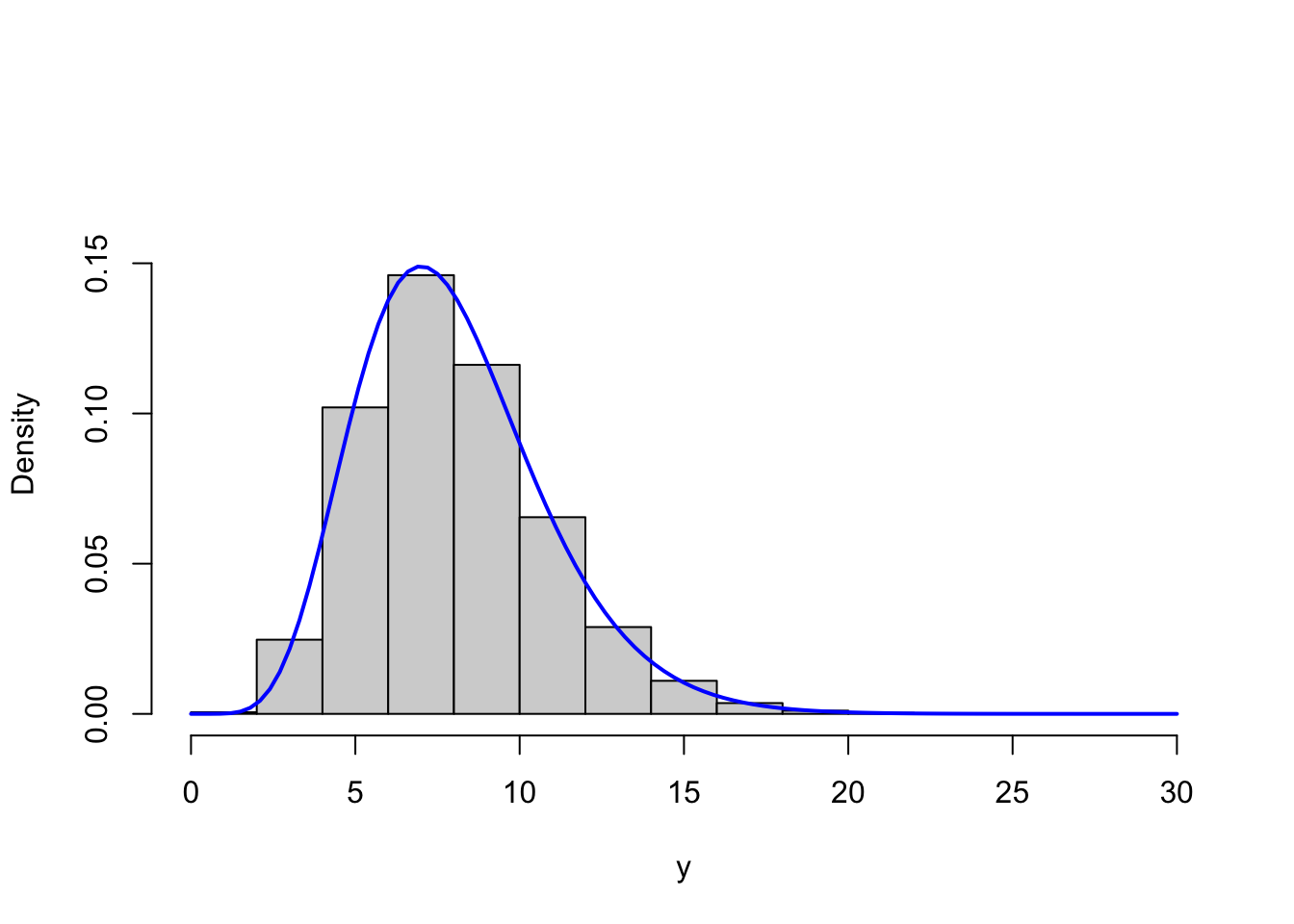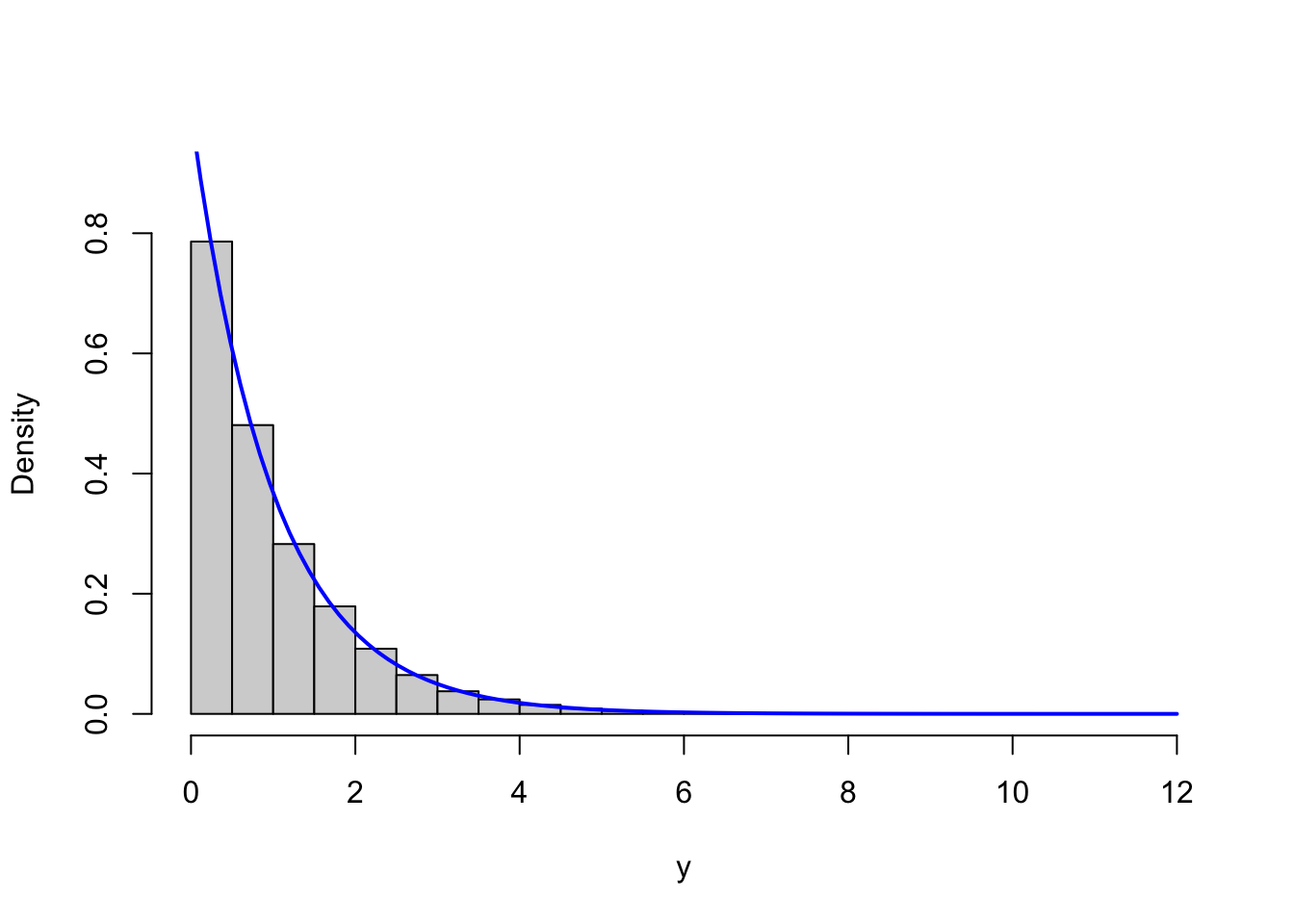Figure 4.4: Histograms of simulated gamma distributed variables with shape parameters $$r = 8$$ (left) and $$r = 1$$ (right) with corresponding theoretical densities (blue).

Though this is only a simple and informal test, it indicates that the implementation correctly simulates from the gamma distribution.

Rejection sampling can be computationally expensive if many samples are rejected. A very tight envelope will lead to fewer rejections, while a loose envelope will lead to many rejections. Using the tracing option as implemented we obtain estimates of the rejection probability and thus a quantification of how tight the envelope is.

y <- gammasim(100000, 16, trace = TRUE)
y <- gammasim(100000, 8, trace = TRUE)
y <- gammasim(100000, 4, trace = TRUE)
y <- gammasim(100000, 1, trace = TRUE)
## r = 16 : 0.00161738
## r = 8 : 0.003745915
## r = 4 : 0.007966033
## r = 1 : 0.04789108

We observe that the rejection frequencies are small with $$r = 1$$ being the worst case with around 5% rejections. For the other cases the rejection frequencies are all below 1%, thus rejection is rare.

A visual comparison of $$q$$ to the (unnormalized) Gaussian density also shows that the two (unnormalized) densities are very close except in the tails where there is very little probability mass.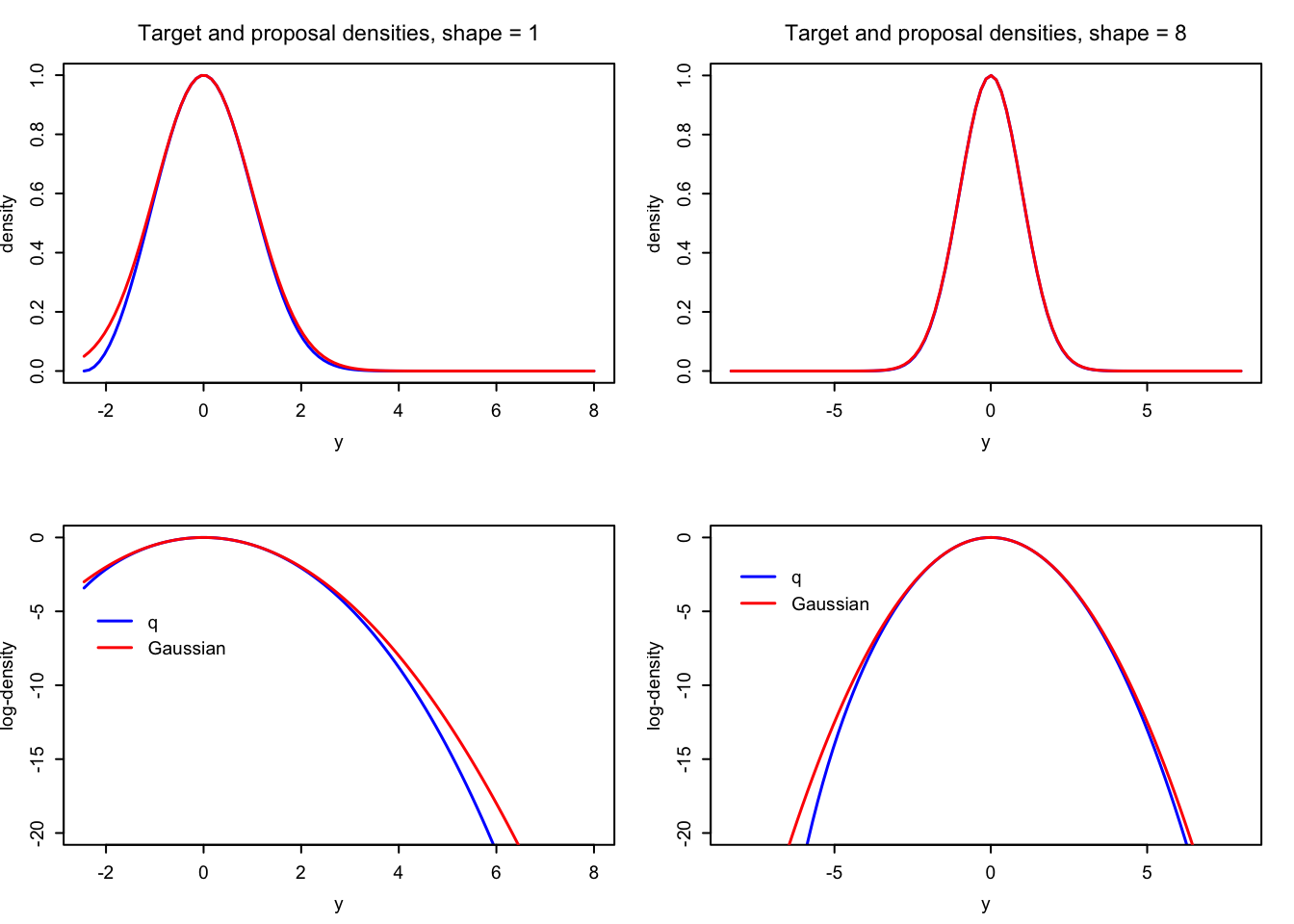Figure 4.5: Comparisons of the Gaussian proposal (red) and the target density (blue) used for eventually simulating gamma distributed variables via a transformation.

### References

Marsaglia, George, and Wai Wan Tsang. 2000. “A Simple Method for Generating Gamma Variables.” ACM Trans. Math. Softw. 26 (3): 363–72. https://doi.org/10.1145/358407.358414.